# Comparing Numbers Greater than Less than Equal to

• Slides: 15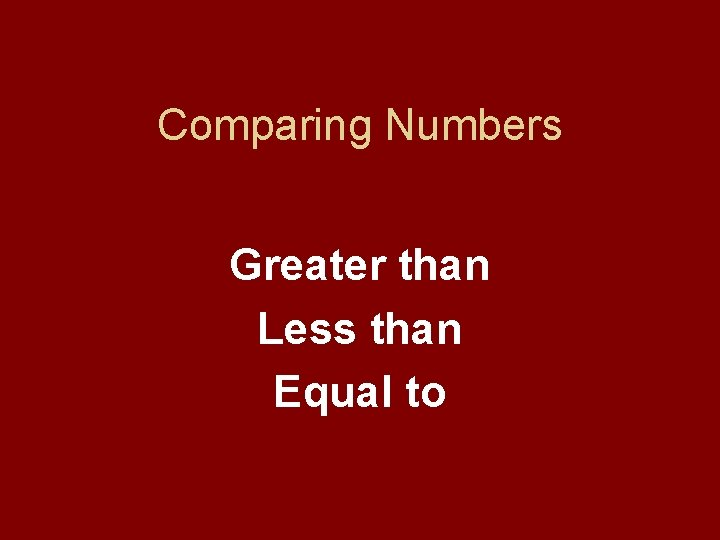Comparing Numbers Greater than Less than Equal to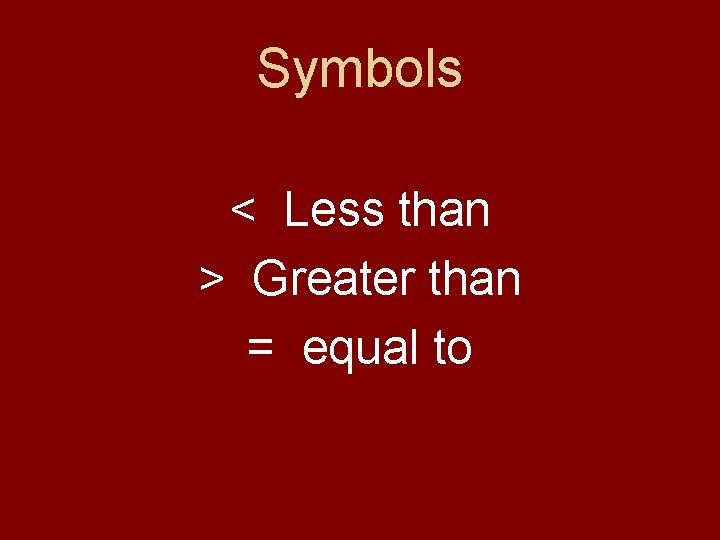Symbols < Less than > Greater than = equal toLook at and read your numbers. Look to see which number has more tens. The one with more tens is the greater number!What if the tens are the same? If the tens are equal… Look at the ones to see which number has the greater ones. The number with the greater ones is greater.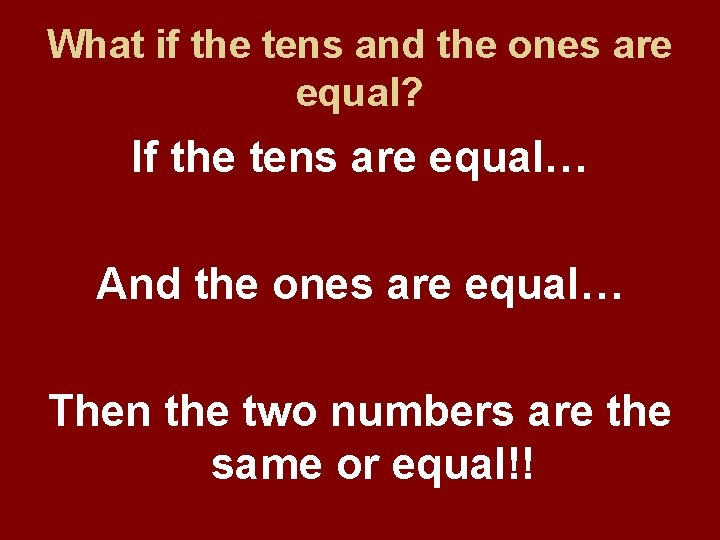What if the tens and the ones are equal? If the tens are equal… And the ones are equal… Then the two numbers are the same or equal!!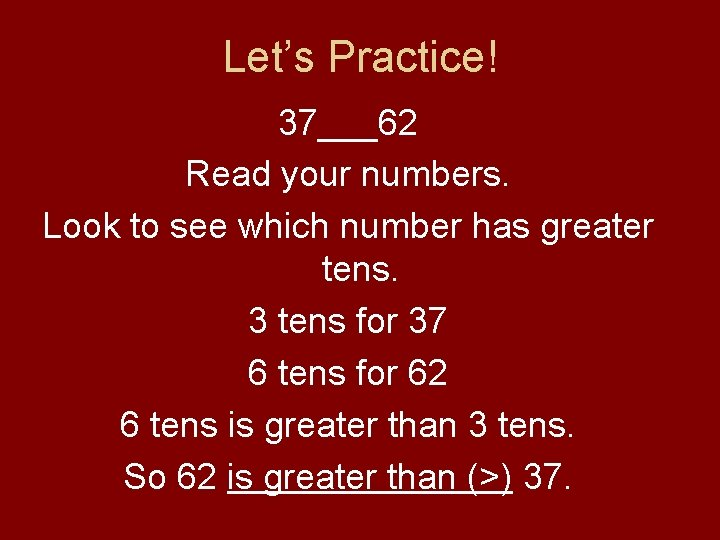Let’s Practice! 37___62 Read your numbers. Look to see which number has greater tens. 3 tens for 37 6 tens for 62 6 tens is greater than 3 tens. So 62 is greater than (>) 37.How about this one? 45___73 Read your numbers. Look to see which number has greater tens. 4 tens for 45 7 tens for 73 7 tens is greater than 4 tens. So 45 is less than (<) 73.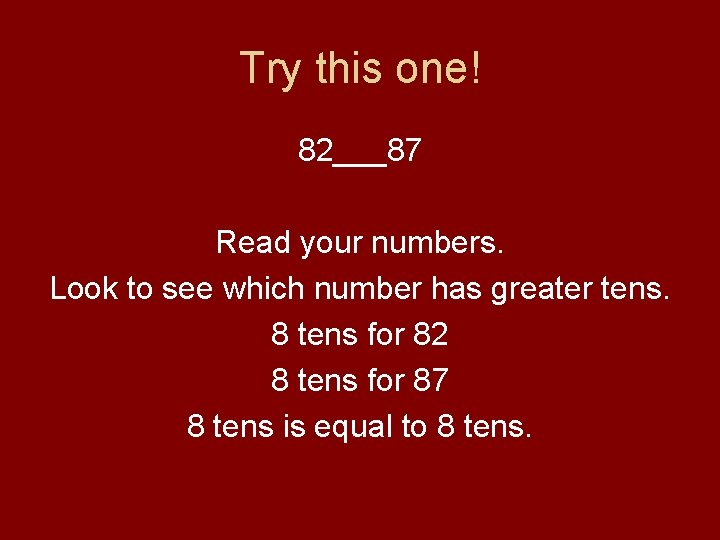Try this one! 82___87 Read your numbers. Look to see which number has greater tens. 8 tens for 82 8 tens for 87 8 tens is equal to 8 tens.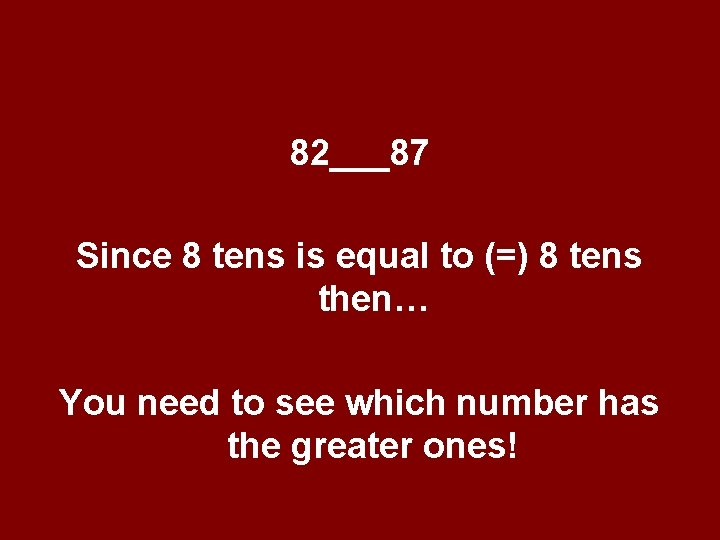82___87 Since 8 tens is equal to (=) 8 tens then… You need to see which number has the greater ones!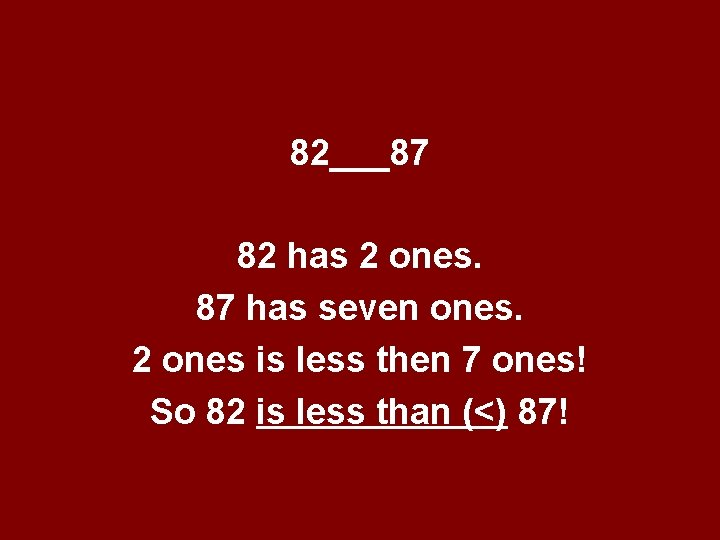82___87 82 has 2 ones. 87 has seven ones. 2 ones is less then 7 ones! So 82 is less than (<) 87!Last one! 57___57 Read both numbers. Look to see which has the greater amount of tens. 5 tens in 57. 5 tens and 5 tens are equal.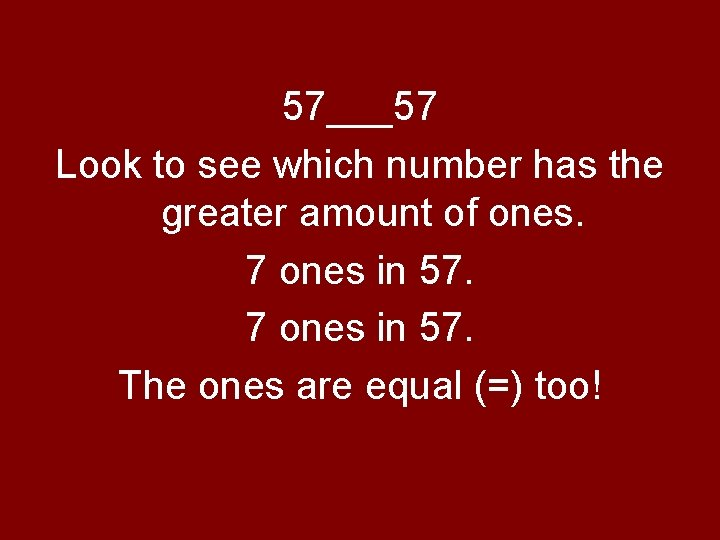57___57 Look to see which number has the greater amount of ones. 7 ones in 57. The ones are equal (=) too!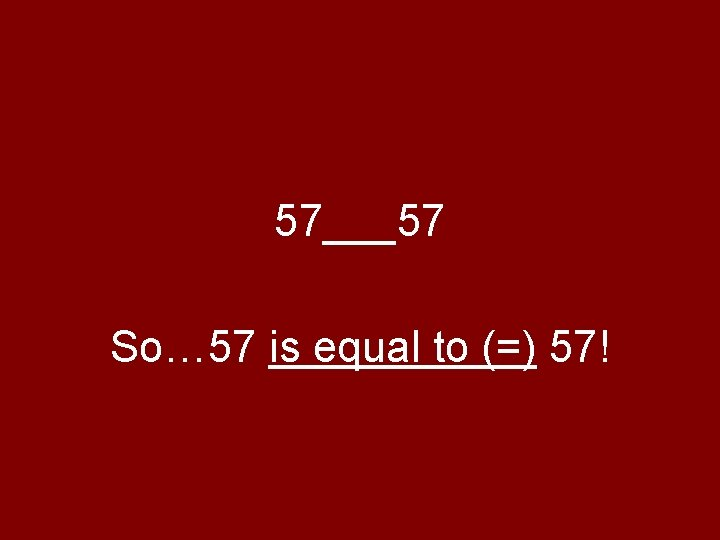57___57 So… 57 is equal to (=) 57!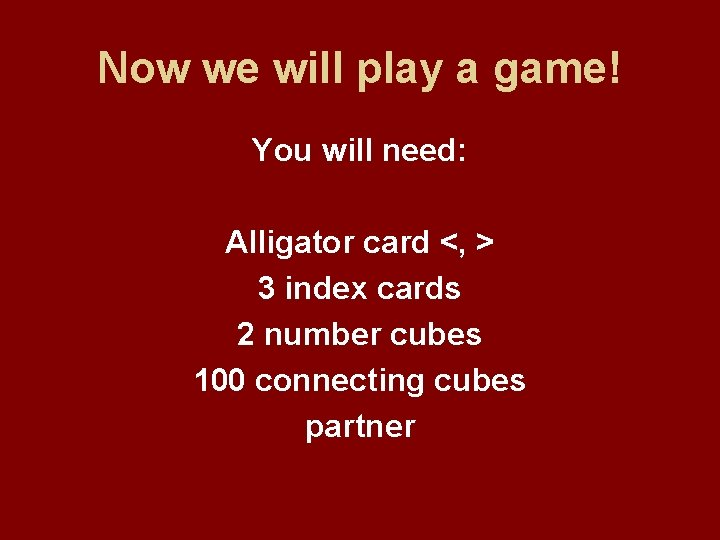Now we will play a game! You will need: Alligator card <, > 3 index cards 2 number cubes 100 connecting cubes partner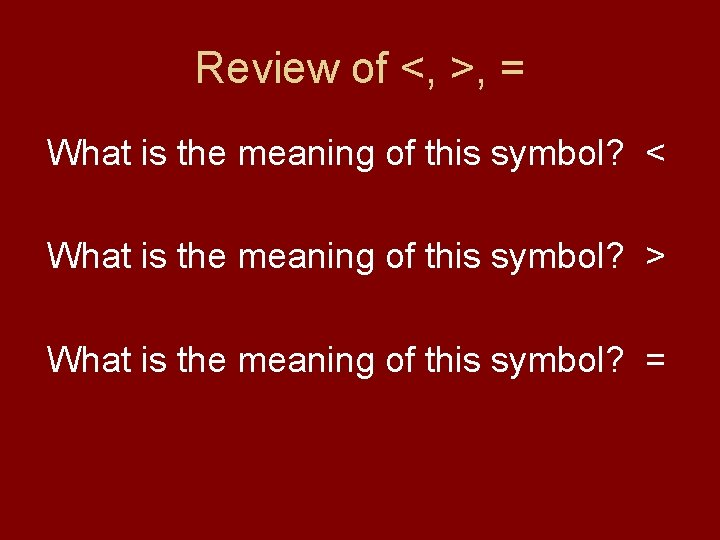Review of <, >, = What is the meaning of this symbol? < What is the meaning of this symbol? > What is the meaning of this symbol? =